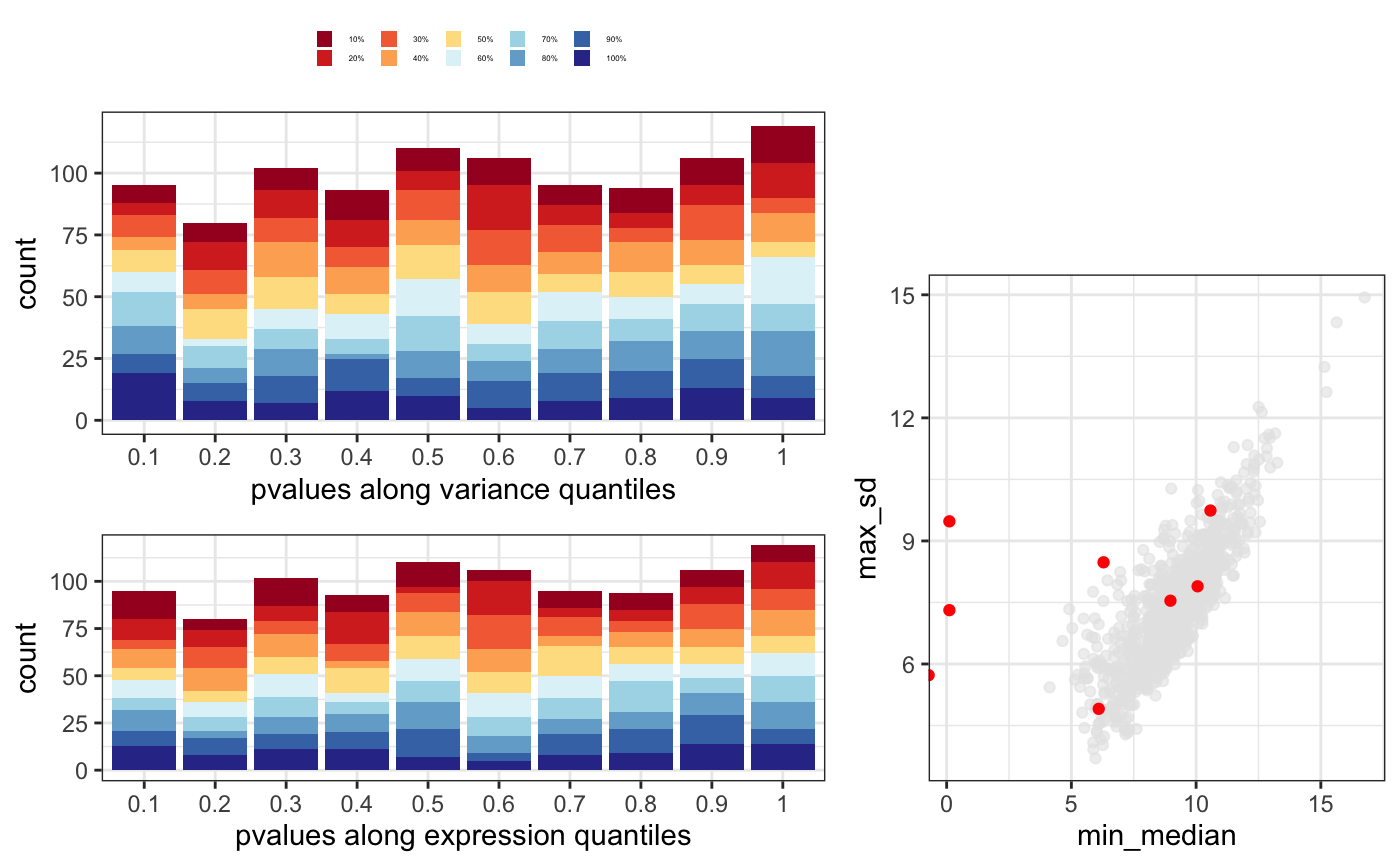This function joins the output of degMean, degVar and degMV in a single plot. See these functions for further information.

degQC(counts, groups, object = NULL, pvalue = NULL)

## Arguments

counts Matrix with counts for each samples and each gene. Character vector with group name for each sample in the same order than counts column names. DEGSet oobject. pvalues of DEG analysis.

ggplot2 object

## Examples

data(humanGender)
library(DESeq2)
idx <- c(1:10, 75:85)
dds <- DESeqDataSetFromMatrix(assays(humanGender)[][1:1000, idx],
colData(humanGender)[idx,], design=~group)
dds <- DESeq(dds)#> using pre-existing size factors#> estimating dispersions#> gene-wise dispersion estimates#> mean-dispersion relationship#> final dispersion estimates#> fitting model and testing#> -- replacing outliers and refitting for 1 genes
#> -- DESeq argument 'minReplicatesForReplace' = 7
#> -- original counts are preserved in counts(dds)#> estimating dispersions#> fitting model and testingres <- results(dds)
degQC(counts(dds, normalized=TRUE), colData(dds)[["group"]],
pvalue = res[["pvalue"]])